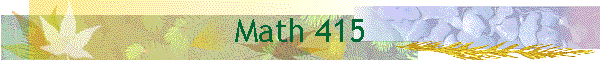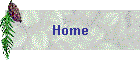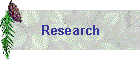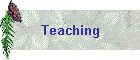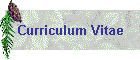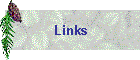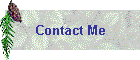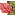Syllabus: Click here for details in a PDF fileCourse: Math 415, Ordinary and Partial Differential Equations, MWF 3:30pm, Pomerene HallTexts: (1) Elementary Differential Equations & Boundary Value Problems (8th Ed) by Boyce and DiprimaTopics: Solutions to first order, second order and higher order ordinary differential equations and partial differential equations; Phase plane analysis; Fourier series; Boundary value problems.Office Hours: MW 4:30-5:30pm @MW410  and by appointmentGrading: first midterm (Mon. Apr 19, 20%), second midterm (Mon. May. 17, 25%), final exam( Thur. Jun 10 3:30pm,30%), class participation (5%), and homework and quiz (20%). The letter grade will be with an approximately 90(A)-80(B)-70(C)-60(D) scale.Policy: NO make-up exams without doctor's excuse. Late Homework will be no credit.Tentative Schedule: updated regularly

The HW problems need to be turned in. HW due every Thursday in TA section. It includes all assignments given in the previous week (Mon, Wed, Fri).

 Monday Wednesday Friday Mar 29 (Spring Classes Begin) Chapter 1: Introduction Chapter 1.2: Solutions of some ODEs HW: 1.1 (11), 1.2(11) Mar 31 Chapter 1.2: Solutions of some ODEs Chapter 1.3: Classification of DEs HW: 1.3(2,3,13,22,23,28) Apr 2 Chapter 2.1 & 2.2 HW:2.1 (6,10,15,18,28) HW 2.2(4,11,25,33) Linear Equation: Method of Integrating Factors Separable Equations Chapter 2.2 Separable Equations Apr 5 Chapter 2.2 Separable Equations Chapter 2.3 Modeling with First Order Equations HW 2.3(4,9,14,23) Apr 7 Chapter 2.3 Modeling with First Order Equations Chapter 2.4 Differences Between Linear and Nonlinear Equations Apr 9 Chapter 2.4 HW: 2.4(11,22,28) Differences Between Linear and Nonlinear Equations Apr 12 Chapter 2.5 Autonomous Equations and Population Dynamics HW 2.5:(3,7,9,17) Apr 14 Chapter 2.5 Autonomous Equations and Population Dynamics 3.1 Homogeneous Equations with Constant Coefficients Apr 16 3.1 Homogeneous Equations with Constant Coefficients HW: 3.1 (6 13 19 20) Apr 19 Chapter 3.2 Fundamental solutions, linear independence HW: 3.2 (2,8,11,13,23) Apr 21 First Exam: Ch1 and Ch2 to 2.5 Apr 23 Chapter 3.3: the Wronskian HW: 3.3 (3,4,15,16,20) Apr 26 Chapter 3.4 Complex Roots of the Characteristic Equation HW: 3.4 (2,3,21) Apr 28 Chapter 3.5 HW:3.5(3,11,12,20,25,30) Repeated Roots; Reduction of Order Apr 30 Chapter 3.6 Nonhomogeneous equations: method of undetermined coefficients HW:3.6 (3,8,14,24) May 3 Chapter 3.8 Mechanical and electrical vibrations HW:3.8 (2,6,9,13) May 5 Chapter 3.9 Mechanical and electrical vibrations HW 3.9 (5,6,7(a,c),10) May 7 Chapter 10.1,Two-Point Boundary Value Problems HW: 10.1 (2,3,4,14,16) May 10 Chapter 10.2, Fourier Series HW: 10.2 (3,4,15,18) May 12 Chapter 10.3 The Fourier Convergence Theorem HW: 10.3 (2,3) May 14 Chapter 10.4 Even and Odd Functions HW: 10.4 (3,6,7,15,18,35) May 17 Chapter 10.5: Heat equation with zero boundary conditions HW 10.5: 3,5,8,10 May 19 Second Exam Chapter 3 and 10.1-10.2 May 21 Chapter 10.6: Other Heat Conduction Problems HW10.6: 2,3,9(a) May 24 Chapter 10.7 Wave equation HW 10.7: 1(a),6(a) May 26 Chapter 10.7,8 Wave equation, Laplace’s equation HW 10.8: 1(a,b),2,5 May 28 Chapter 10.8, Chapter 7.1 Laplace’s equation,Systems of first order equations: Linearization at equilibrium – the problem of stability HW 7.1: 2,5 May 31 (Memorial Day - no class) June 2 Chapter 7.3, ex 4, 7,4 Thm1, 2 , 7.5 Eigenvalues, eigenvectors, General solution of X' = PX, homogeneous liear systems with constant coefficients HW 7.3(15,17,19), 7.5 (1,4) June 4  Chapter 7.4-7.5: Homogeneous linear systems with constant coefficients   Chapter 7.6: Complex eigenvalues HW: 7.6 (2,6) Review June 7 Final exam week June 9 Final exam week June 11 Final exam week Spring commencement